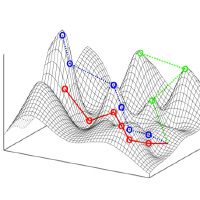#MDEOptimiser

MDEOptimiser now supports the generation of atomic consistency preserving search operators(aCPSOs). aCPSOs are mutation operators that ensure multiplicity constraint satisfaction after their application.

A description of the currently supported search operators is given in the two sections below. The first section shows node manipulation patterns supported and the second includes edge manipulation cases supported.

The two tables below assume the multiplicity pattern between two nodes as shown in the figure below.## Node Manipulation

A list of the supported node manipulation multiplicities is included in the tables below. The first table shows the node creation operators. The second table contains the node deletion operators.

Create Node n=0 n=1 and m > n n > 1 and m > n n = m
k >= 0
l > k
l < *
c A Create A add n B (f#l A)
Create A lb r single B
Create A lb r single B
Create A lb r many B
Create A add n B (f#l A)
k >= 0
l = *
k = l   Create A lb r single B Create A add n B
Create A lb r single B
Create A lb r many B
N/A
Delete Node m > n and m < * m = * n = m
k = 0 Delete A
k > 0
l > k
Delete A (require each B still has #k A)
k=l=1 Delete A r lb sg B (f#m A) Delete A r lb sg B N/A
k=l > 1 Delete A r lb sg B (f#m A)
d A r lb mm B (f#m A)
Delete A r lb sg B
d A r lb mm B
N/A

## Edge Manipulation

The list of supported multiplicities for generating edge manipulation search operators is included in the tables below. The first table contains the edge creation supported multiplicities and the generated operators. The second table contains the operators generated for edge removal.

Edge Creation m < * m = * n=m
l < * Add edge NAC A B Add edge NAC B Swap edge